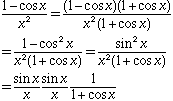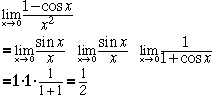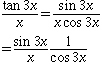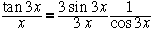Subject: Two limits How do I find lim (1-cosx)/(x^2) as x-> 0 and lim (tan3x)/x as x->0 Hi, Many of the limits in first semester calculus that involve trig functions revolve around one fundamental fact. That fact isThe problem then usually involves some algebraic and/or trigonometric manipulation to use this fact. As is often the case in algebra and trigonometry you need to see a pattern somewhere.    In your first problem the numerator is 1 - cos x and I know that 1 - cos2 x = sin2 x. I also know that 1 - cos2 x = (1 - cos x)(1 + cos x) and hence I can proceed as follows.ThusIn your second problem tan3x = sin3x/cos3x and thusFor the first factor to be of the form sint/t so that you can apply the fact mentioned above you need t = 3x so multiply the numerator and denominator by 3 to getNow take the limit as x->0. Cheers, Harley

Go to Math Central